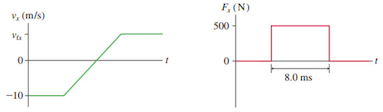Problem: A 270 g ball collides with a wall. The figures show the ball's velocity and the force exerted on the ball by the wall. What is vfx, the balls rebound velocity?

FREE Expert Solution
92% (144 ratings)
Problem Details

A 270 g ball collides with a wall. The figures show the ball's velocity and the force exerted on the ball by the wall. What is vfx, the balls rebound velocity?